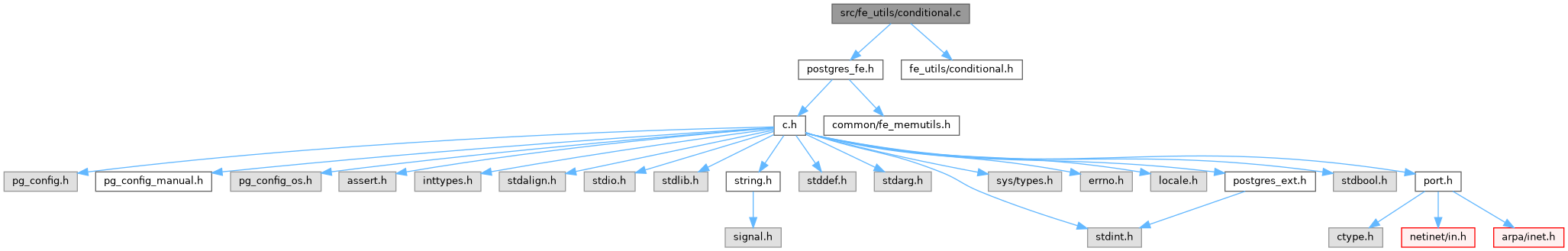PostgreSQL Source Code  git master
conditional.c File Reference
`#include "postgres_fe.h"`
`#include "fe_utils/conditional.h"`
Include dependency graph for conditional.c:Go to the source code of this file.

## Functions

ConditionalStack conditional_stack_create (void)

void conditional_stack_reset (ConditionalStack cstack)

void conditional_stack_destroy (ConditionalStack cstack)

void conditional_stack_push (ConditionalStack cstack, ifState new_state)

bool conditional_stack_pop (ConditionalStack cstack)

int conditional_stack_depth (ConditionalStack cstack)

ifState conditional_stack_peek (ConditionalStack cstack)

bool conditional_stack_poke (ConditionalStack cstack, ifState new_state)

bool conditional_stack_empty (ConditionalStack cstack)

bool conditional_active (ConditionalStack cstack)

void conditional_stack_set_query_len (ConditionalStack cstack, int len)

int conditional_stack_get_query_len (ConditionalStack cstack)

void conditional_stack_set_paren_depth (ConditionalStack cstack, int depth)

int conditional_stack_get_paren_depth (ConditionalStack cstack)

## ◆ conditional_active()

 bool conditional_active ( ConditionalStack cstack )

Definition at line 140 of file conditional.c.

141 {
142  ifState s = conditional_stack_peek(cstack);
143
144  return s == IFSTATE_NONE || s == IFSTATE_TRUE || s == IFSTATE_ELSE_TRUE;
145 }
ifState conditional_stack_peek(ConditionalStack cstack)
Definition: conditional.c:106
ifState
Definition: conditional.h:30
@ IFSTATE_ELSE_TRUE
Definition: conditional.h:40
@ IFSTATE_TRUE
Definition: conditional.h:32
@ IFSTATE_NONE
Definition: conditional.h:31

References conditional_stack_peek(), IFSTATE_ELSE_TRUE, IFSTATE_NONE, and IFSTATE_TRUE.

## ◆ conditional_stack_create()

 ConditionalStack conditional_stack_create ( void )

Definition at line 18 of file conditional.c.

19 {
21
23  return cstack;
24 }
void * pg_malloc(size_t size)
Definition: fe_memutils.c:47
Definition: conditional.h:68

Referenced by CheckConditional(), main(), and MainLoop().

## ◆ conditional_stack_depth()

 int conditional_stack_depth ( ConditionalStack cstack )

Definition at line 84 of file conditional.c.

85 {
86  if (cstack == NULL)
87  return -1;
88  else
89  {
91  int depth = 0;
92
93  while (p != NULL)
94  {
95  depth++;
96  p = p->next;
97  }
98  return depth;
99  }
100 }
struct IfStackElem * next
Definition: conditional.h:63

## ◆ conditional_stack_destroy()

 void conditional_stack_destroy ( ConditionalStack cstack )

Definition at line 43 of file conditional.c.

44 {
46  free(cstack);
47 }
void conditional_stack_reset(ConditionalStack cstack)
Definition: conditional.c:30
#define free(a)

References conditional_stack_reset(), and free.

Referenced by CheckConditional(), main(), and MainLoop().

## ◆ conditional_stack_empty()

 bool conditional_stack_empty ( ConditionalStack cstack )

Definition at line 130 of file conditional.c.

131 {
133 }

## ◆ conditional_stack_get_paren_depth()

 int conditional_stack_get_paren_depth ( ConditionalStack cstack )

Definition at line 184 of file conditional.c.

185 {
186  if (conditional_stack_empty(cstack))
187  return -1;
189 }
bool conditional_stack_empty(ConditionalStack cstack)
Definition: conditional.c:130
int paren_depth
Definition: conditional.h:62

## ◆ conditional_stack_get_query_len()

 int conditional_stack_get_query_len ( ConditionalStack cstack )

Definition at line 162 of file conditional.c.

163 {
164  if (conditional_stack_empty(cstack))
165  return -1;
167 }

## ◆ conditional_stack_peek()

 ifState conditional_stack_peek ( ConditionalStack cstack )

Definition at line 106 of file conditional.c.

107 {
108  if (conditional_stack_empty(cstack))
109  return IFSTATE_NONE;
111 }
ifState if_state
Definition: conditional.h:60

## ◆ conditional_stack_poke()

 bool conditional_stack_poke ( ConditionalStack cstack, ifState new_state )

Definition at line 118 of file conditional.c.

119 {
120  if (conditional_stack_empty(cstack))
121  return false;
123  return true;
124 }

## ◆ conditional_stack_pop()

 bool conditional_stack_pop ( ConditionalStack cstack )

Definition at line 69 of file conditional.c.

70 {
72
73  if (!p)
74  return false;
76  free(p);
77  return true;
78 }

## ◆ conditional_stack_push()

 void conditional_stack_push ( ConditionalStack cstack, ifState new_state )

Definition at line 53 of file conditional.c.

54 {
55  IfStackElem *p = (IfStackElem *) pg_malloc(sizeof(IfStackElem));
56
57  p->if_state = new_state;
58  p->query_len = -1;
59  p->paren_depth = -1;
62 }

## ◆ conditional_stack_reset()

 void conditional_stack_reset ( ConditionalStack cstack )

Definition at line 30 of file conditional.c.

31 {
32  if (!cstack)
33  return; /* nothing to do here */
34
35  while (conditional_stack_pop(cstack))
36  continue;
37 }
bool conditional_stack_pop(ConditionalStack cstack)
Definition: conditional.c:69

References conditional_stack_pop().

## ◆ conditional_stack_set_paren_depth()

 void conditional_stack_set_paren_depth ( ConditionalStack cstack, int depth )

Definition at line 173 of file conditional.c.

174 {
177 }
Assert(fmt[strlen(fmt) - 1] !='\n')

Referenced by save_query_text_state().

## ◆ conditional_stack_set_query_len()

 void conditional_stack_set_query_len ( ConditionalStack cstack, int len )

Definition at line 151 of file conditional.c.

152 {